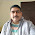## Wednesday, October 10, 2012

### Sound Frequency

*sound science series # 2
Frequency:
Frequency tells us how frequently an event occurs. Suppose you are beating a drum. How many times you are beating the drum per unit time is called the frequency of your beating the drum.
The change in density from the maximum value to the minimum value, again to the maximum value, makes one complete oscillation.
The number of such oscillations per unit time is the frequency of the sound wave.

Sound Frequency:
• Sound is a mechanical wave that is an oscillation of pressure transmitted through a solid, liquid, or gas, composed of frequencies within the range of hearing.
• The number of cycles per unit of time is called the frequency
• For convenience, frequency is most often measured in cycles per second (cps) or the interchangeable Hertz (Hz) (60 cps = 60 Hz), named after the 19th C. physicist. 1000 Hz is often referred to as 1 kHz (kilohertz) or simply '1k' in studio parlance.

Human range of sound frequency:  The range of human hearing in the young is approximately 20 Hz to 20 kHz—the higher number tends to decrease with age (as do many other things). It may be quite normal for a 60-year-old to hear a maximum of 16,000 Hz.
For comparison, it is believed that many whales and dolphins can create and perceive sounds in the 175 kHz range. Bats use slightly lower frequencies for their echo-location system.

Above and below frequency: Frequencies above and below the range of human hearing are also commonly used in computer music studios. We refer to these ranges as:
 <20 Hz 20-20kHz >20kHz sub-audio rate audio rate ultrasonic
Sub-audio signals are used as controls (since we can't hear them) in synthesis to produce effects like vibrato. The lowest 32' organ pipes also produce fundamental frequencies below our ability to hear them (the lowest, C four octaves below 'middle C' is 16.4 Hz) — we may sense the vibrations with our body or extrapolate the fundamental pitch from the higher audible frequencies (discussed below), but these super-low ranks are usually doubled with higher ranks which reinforce their partials.

Frequency and wavelength:  Frequency is directly related to wavelength, often represented by the Greek lambda (). The wavelength is the distance in space required to complete a full cycle of a frequency. The wavelength of a sound is the inverse of its frequency. The formula is:
wavelength () = speed of sound/frequency
 Example: A440 Hz (the frequency many orchestras tune to) in a dry, sea level, 68°F room would create a waveform that is ~2.5 ft. long (2.56 = 1128 (feet/sec) / 440). Be certain to measure the speed of sound and wavelength in the same units. Notice how if the speed of sound changed due to temperature, altitude, humidity or conducting medium, so too would the wavelength.
Low frequency longer wavelength: As can be seen from the above formula, lower frequencies have longer wavelengths. We are able to hear lower frequencies around a corner because the longer wavelengths refract or bend more easily around objects than do shorter ones. Longer wavelengths are harder for us to directionally locate, which is why you can put your Surround Sound subwoofer most anywhere in a room except perhaps underneath you. At 20°C, sound waves in the human hearing spectrum have wavelengths from 0.0172 m (0.68 inches) to 17.2 meters (56.4 feet).

Doppler effect or Doppler shift: One particularly interesting frequency phenomenon is the Doppler effect or Doppler shift. You've no doubt seen movies where a police siren or train whistle seems to drop in pitch as it passes the listener. In actuality, the wavelength of sound waves from a moving source are compressed ahead of the source and expanded behind the source, creating a sensation of a higher and then lower frequency than is actually being produced by the source. This is the same phenomenon used by astronomers with light wavelengths to calculate the speed and distance of a receding star. The light wavelengths as stars move away are shifted toward the red end of the spectrum, hence the term red shift.

Formulas and equations for sound: c = λ × f        λ = c / f = c × T        f = c / λ
 Physical value symbol unit formula frequency f = 1/T Hz = 1/s f = c / λ wavelength λ m λ = c / f time period or cycle duration T = 1/f s T = λ / c wave speed c m/s c = λ × f

Wave frequency in Hz = 1/s and wavelength in nm = 10−9 m

#### 1 comment:

1.Sound Healing therapy moves the patient closer to health, harmony and state of balance. There are number of frequencies which heal the illness in a natural and effective way which is known as Healing Sound. Listening to the specially recorded sound frequencies during your daily routine can create the positive changes which will enhance the quality of your life.